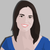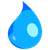# Calculation for Hydrant flow rate

564
7
04-14-2022 01:05 PMby
Occasional Contributor III

I have a Survey123 form which my Hydrant inspection guys use when they go out inspecting Hydrants. What I want to do in Survey 123 is be able to calculate the flow rate for each hydrant but I am not sure how to enter this into the XLS form. They already are collecting data on the Static Pressure, Residual Pressure, PSI and Diameter. Can someone help me with the formula to calculate flow rate and where to enter it in the XLS form.
I assume it goes under the Calculate field but am not sure.

Thanks

Tags (3)
1 Solution

Accepted SolutionsbyEsri Notable Contributor

Not an expert on this, but I found potential explanation here: https://blog.qrfs.com/240-pitot-gauges-how-do-i-calculate-the-psi-to-gpm-conversion/#pitot-calculati...

Following the formula in the blog above, the attached XLSForm would do the trick.

7 RepliesbyMVP Regular Contributor

I could try to help with the Survey123 syntax (and yes, you'll put the expression in the Calculate column), but could you explain what mathematical formula you need to use to determine flow rate?

Best,
Katherineby
Occasional Contributor III

Thanks for the response!

I'm not sure if you could provide me with the formula for flow rate. I saw one when I tried searching for it and thought maybe there is a standard formula that is used and maybe if I asked someone would reply with it. I have someone else here working on trying to get it for me too. That's not the main question I had though.

Once I get this formula how would I enter it on the survey123 form. Should I use 'calculate' as type and then Flow Rate as name and label? Should the expression just be entered as is once I know what the expression is?

ThanksbyEsri Notable Contributor

Not an expert on this, but I found potential explanation here: https://blog.qrfs.com/240-pitot-gauges-how-do-i-calculate-the-psi-to-gpm-conversion/#pitot-calculati...

Following the formula in the blog above, the attached XLSForm would do the trick.by
Occasional Contributor III

Thanks for that link! That's what I was looking forby
Occasional Contributor III

I have added these fields and this calculation into my Survey123 form and the form opens in the Survey123 app like I wanted. Will the GPM field calculate once I submit the form?byEsri Contributor

When running the referenced calculation above, keep in mind a few variables that may impact results

• the hydrants being tested may vary across the network (old, new, size and type varies etc), as would the diameter inside of the hydrant based on each different type, that could impact the results in the equation, and lead to more complex drop downs etc.
• as a hydrant is flowed, pressure in the water network (P) likely decreases, there by reducing the Q that the formula is solving form.  Thus if flow varies/decreases, which total volume would you seek to have entered/recorded?

To not get too worried about the detail if you don't need to, how accurate of a number do you need to report?  I have seen everything from some utilities putting flow meters on the hydrant to measure the flow during tests (ideal but takes time and requires flow meters), all the way to to stop-stop-watching the flow event and assuming a flow rate (i.e. 500gpm or some avg based on the size of hydrant).  Then multiply by the minutes flowed to arrive at the total volume flushed.  If you have time, it could be worth confirming accuracy of calculation against the accuracy of the number you need to have entered:  does the additional level of effort provide value.by
Occasional Contributor III